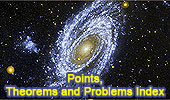# Geometry: Points, Theorems and Problems - IndexEuclid's Elements Book I, 23 Definitions. One-page visual illustration.
Point: Definition 1, Book I
Euclid's Elements Book. Index

Meanings and syntactic of 'POINT'.
Created using Wordflex Touch Dictionary for iPad in association with Oxford University Press.

Collinear Points.
Theorems and Problems

Midpoints.
Theorems and Problems

Geometry Problem 1352.
Rectangles, Sum of Squared Distances.

Angles.
Endpoint, Vertex.

Perpendicular lines.
Intersection point.

Triangles.
Non-collinear points. Vertices.

Altitude Index.
Point: Foot of the altitude.

Median Index.
Mid-point.

Concyclic Points.

Circles
Center point.

Intersecting Circles Index.
Point of intersections

Point of tangency.

Tangent Circles Index
Point of tangency.

Triangle Centers.
Center points

Centroid /Barycenter. Index.
Point where the three medians meet.

Circumcenter. Index.
Point where the three perpendicular bisectors meet.

Incenter. Index.
Point where the three angle bisectors meet.

Excenter. Index.